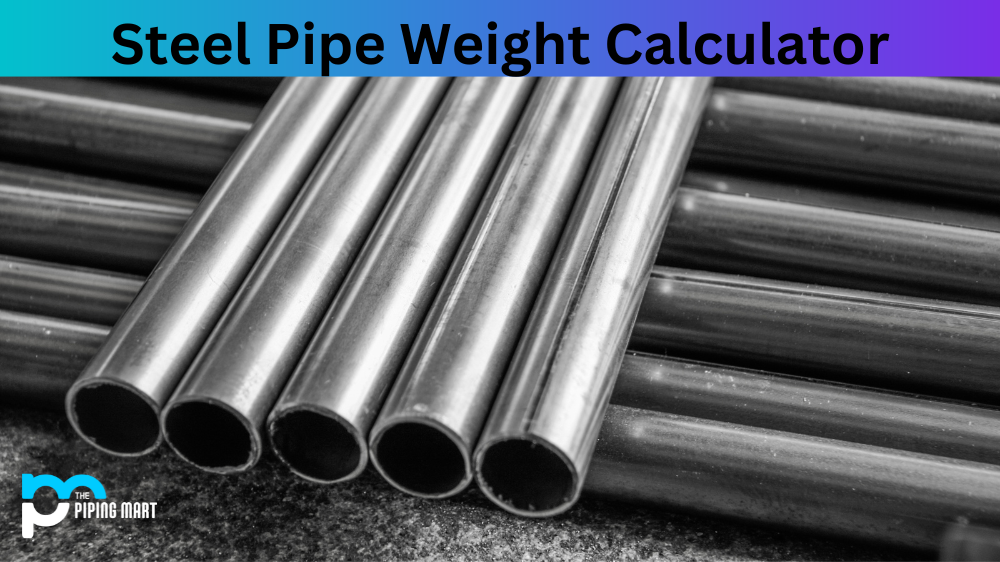ThePipingMart Blog Weight Calculator 316 stainless steel weight calculator

# 316 stainless steel weight calculator## Stainless Steel Bar Weight Calculator, SS Round Bar Weight Calculator, SS Hex Bar Weight Calculator, SS 316 Round Bar Weight Calculator, SS Bar Weight Calculator, SS 316 Round Bar Weight Calculator, SS Round Bar Weight Calculation Formula, SS Round Weight Calculator

An ss316 weight calculator can be a valuable tool for anyone looking to find out the exact weight of ss316 or ss316l metal components. Creating custom ss316 or ss316l parts can be quite complex, but with an ss316 weight calculator, it’s easy to get an accurate estimation of the amount of steel needed to complete the job. The ss316 weight calculator is especially helpful if you don’t have an estimating guide handy, and it offers much more precision than manual measurements.

Results

It can also save time and money in industrial projects that require stainless steel parts. Everyone working on ss316 or ss316l steel-based projects should make sure they have access to an ss316L weight calculator so they are well prepared for any project, no matter how complex.

### Related Post## Steel Pipe Weight CalculatorSteel Pipe Weight Calculator

Steel Pipe Weight Calculator, Steel Pipe Weight Calculator Metric, Steel Pipe Weight Formula. Steel Pipe Weight Calculator If you are in the market for some## Aluminium Strip / Flat Weight CalculatorAluminium Strip / Flat Weight Calculator

Aluminum Square Tube Weight Calculator, Aluminum Rectangular Tube Weight Calculator, Aluminium Rectangular Tube Weight Calculator. How to calculate aluminium weight? Aluminium Strip Weight Calculation One## Aluminium Section/Beam Weight CalculatorAluminium Section/Beam Weight Calculator

Aluminium Hollow Section Weight Calculator, Aluminium Rectangular Hollow Section Weight Calculator, Aluminium Section Weight Calculator, Aluminum Section Weight Calculator, Aluminum i Beam Weight Calculator, How